## Solution to 1986 Problem 65

 Because the fixed point is far from the loop, we can approximate the current loop is an ideal magnetic dipole. The magnetic field due to an ideal magnetic dipole with dipole moment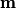$\mathbf{m}$ is given by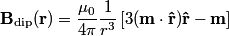\begin{align*}\mathbf{B}_{\text{dip}}(\mathbf{r}) = \frac{\mu_0}{4 \pi} \frac{1}{r^3}\left[3 (\mathbf{m}\cdot \mathbf{\hat{r}...Therefore, if your location relative to the ideal magnetic dipole is held constant, then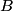$B$ is proportional to the magnetic dipole moment$\mathbf{m}$. The dipole moment of a current loop is given by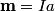\begin{align*}\mathbf{m} = I a\end{align*}where I is the current in the loop and a is the area of the loop. The area a for a circular loop of radius b is equal to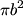$\pi b^2$, so the magnetic dipole moment equals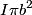$I \pi b^2$, thus the magnetic field is proportional to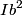$I b^2$. Hence answer (B) is correct.